Sponsored Link •Weblogs Forum
The Adventures of a Pythonista in Schemeland/11

3 replies on 1 page. Most recent reply: Nov 11, 2008 10:00 AM by Michele Simionato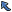Back to Topic List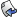Reply to this Topic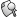Search Forum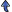Threaded View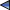Previous Topic Next Topic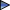Flat View: This topic has 3 replies on 1 page
 Michele Simionato Posts: 222 Nickname: micheles Registered: Jun, 2008
The Adventures of a Pythonista in Schemeland/11 (View in Weblogs)
Posted: Nov 11, 2008 10:00 AMReply
Summary
In this episode I will discuss the multiple evaluation issue, then I will show how macros can improve performance. Finally, I will give a practical example of how macros can be used to define a unit test framework.
 Advertisement# The problem of multiple evaluation

In episode #10 I gave an example of a macro implementing a C-like for loop and I said that it was suffering from the problem of multiple evaluation. Here I explain what the problem is and how to cure it. In order to understand the issue, you must always remember that macros expand code at compile time, but they not evaluate it: this means that pattern variables do not correspond to evaluated expression, as ordinary variables, but they correspond to expressions to be evaluated later, at runtime.

As a consequence, it is easy to write macros that evaluate expressions more times than needed. For instance, consider the following simplified version of a C-like for loop, with a runtime type check:

```(def-syntax (for i start end body ...)
#'(begin
(assert (and (number? start) (number? end))); type-check
(let loop ((i start))
(unless (>= i end) body ... (loop (+ i 1))))))
```

Suppose the variable end to be determined dynamically with a computation:

```> (define (get-end)
(printf "computing the value of end\n")
3)
```

Then our naive macro suffers from the multiple evaluation problem:

```> (for i 0 (get-end) 'do-nothing)
computing the value of end
computing the value of end
computing the value of end
computing the value of end
computing the value of end
```

As you see, in this example end is recomputed 5 times! The reason is clear if you look at the expansion of the macro:

```> (syntax-expand (for i 0 (get-end) 'do-nothing))
(begin
(assert (and (number? 0) (number? (get-end))))
(let loop ((i 0))
(unless (>= i (get-end)) 'do-nothing (loop (+ i 1)))))
```

The get-end function is called once in the assertion and four times in the loop; that is inefficient and can have very dramatic effects if the function has side effects. The solution is to save the value of end (and we could do the same for the value of start, which is computed twice) in a variable:

```(def-syntax (for i start end body ...)
#'(let ((s start) (e end))
(assert (and (number? s) (number? e)))
(let loop ((i s))
(unless (>= i e) body ... (loop (+ i 1))))))
```

Now get-end is called only once and we are all happy :-)

As an exercise, you could extend for to accept a generic step. You can find the solution in the Italian original version of this article, which is quite different and uses syntax-rules.# Taking advantage of multiple evaluation

Sometimes we can make good use of the multiple evaluation "feature". For instance, let me consided again the higher order function call I introduced in episode #5, when discussing benchmark. That function has an issue: it is called at each iteration in the inner loop and therefore it wastes time. However, it is possible to replace the higher order function with a macro, therefore avoiding the cost of a function call. Here is the code for a repeat macro doing the job of call:

```(library (repeat-macro)
(export repeat)
(import (rnrs) (sweet-macros))

(def-syntax (repeat n body body* ...)
#'(let loop ((i 0))
(when (< i n) body body* ... (loop (+ 1 i)))))
)
```

repeat expands into a loop and therefore the body is evaluated n times, which is exactly what we need for a benchmark. To check that the macro is effectively more efficient, I did measure the time spent in summing 1+1 ten million of times:

```(import (rnrs) (repeat-macro) (repeat) (only (ikarus) time))
(define n (string->number (car (reverse (command-line)))))
(time (call 10000000 + 1 n))
(time (repeat 10000000 (+ 1 n)))
```

I took the number n from the command line arguments in order to fool the compiler: if I hard coded (+ 1 1), the compiler would replace it with 2 at compilation time, therefore not performing the computation! (In the original version of this episode I made that mistake, thanks to Aziz Ghuloum for pointing it out). The output of the script is the following:

```\$ scheme-script repeat-benchmark.ss 1
running stats for (call 10000000 + 1 n):
no collections
396 ms elapsed cpu time, including 0 ms collecting
394 ms elapsed real time, including 0 ms collecting
32 bytes allocated
running stats for (repeat 10000000 (+ 1 n)):
no collections
40 ms elapsed cpu time, including 0 ms collecting
40 ms elapsed real time, including 0 ms collecting
0 bytes allocated
```

As you see, avoiding the function call makes a lot of difference (the benchmark is 10 times faster!) since the great majority of the time is wasted in calling the benchmarking function and not in the real addition. Here the improvement is spectacular since summing two integers is a very fast operation: replacing call with repeat in the benchmark factorial does not make a big difference instead.

# A micro-framework for unit tests

It is time to give a more practical example of Scheme macros. In this paragraph, I will define a very simple unit test framework called easy-test.

Clearly, there are already unit test frameworks available for Scheme, including two SRFIs (64 and 78); my interests here is not in the testing framework, it is in the implementation, which makes a pedagogical exercise in macrology.

The source code takes just a page:```(library (easy-test)
(export test run-tests runner run print-nothing print-dot print-msg)
(import (rnrs) (only (ikarus) printf) (sweet-macros))

;; test macro
(def-syntax (test description expr expected)
#'(lambda (cmd)
(case cmd
((descr) description)
((values) '(expr  expected))
((run) (equal? expr expected))
(else (error 'test "Invalid command" cmd)))))

;; three helper functions
(define (print-nothing descr expr expected)
(display ""))

(define (print-dot descr expr expected)
(display "."))

(define (print-msg descr expr expected)
(printf "\n'~a' failed. Expected ~a, got ~a\n" descr expected expr))

;; full runner
(define (run-tests print-success print-failure . tests)
(let loop ((tests tests) (success 0) (failure 0))
(if (null? tests)
(list success failure)
(let* ((test1 (car tests))
(descr (test1 'descr)) (vals (test1 'values)))
(if (test1 'run)
(begin; the test succeeded
(apply print-success descr vals)
(loop (cdr tests) (+ 1 success) failure))
(begin; the test failed
(apply print-failure descr vals)
(loop (cdr tests) success (+ 1 failure))))))))

;; runner factory
(define (runner print-success print-failure)
(lambda tests (apply run-tests print-success print-failure tests)))

;; default runner
(define run (runner print-dot print-msg))

)
```

The core of the framework is the test macro, which is a bit different from the macros we have defined until now. The reason why the test macro is different is that it expands into a lambda-expression and therefore the arguments of the macro are evaluated only when the lambda function is called and not at definition time. In other words, we are using a pattern of delayed evaluation here. This is important, since we want to distinguish the definition of a test from its execution. For instance, let me define a trivial test:

```> (import (easy-test))
> (define test1 (test "1+1=2" (+ 1 1) 2))
```

The first argument of the macro is a string describing the test, which is nice to have in the error message for failed tests; the second argument of the macro is the expression to check and the third argument is the expected result.

Macro application results in a function which is able to respond to the commands 'descr (returning the description string), 'values (returning a list with the quoted input expression and the quoted expected output) and 'run (returning the result of the test, as a boolean flag). This is implemented via the case expression in the test macro:

```(case cmd
((descr) description)
((values) '(expr  expected))
((run) (equal? expr expected))
(else (error 'test "Invalid command" cmd)))
```

Here is how it works in our example:

```> (test1 'descr)
"1+1=2"
> (test1 'values)
((+ 1 1) 2)
> (test1 'run) ; the test passed
#t
```

The framework provides three predefined functions print-nothing, print-msg and print-dot to print feedback about how the tests are going; moreover, it is possible to define custom reporting functions. A reporting function is simply a function with three arguments (descr expr expected) where descr is a string with the description of the test, expr is the expression to be checked and expected is the expected result. You can specify the reporting functions to use by defining a test runner as in this example:

```> (define run-quiet (runner print-nothing print-msg))
> (run-quiet
(test "1+1=2" (+ 1 1) 2)
(test "2*1=2" (* 2 1) 2)
(test "2+2=3" (+ 2 2) 3))
'2+2=3' failed. Expected 3, got 4
(2 1)
```

The runner returns a list with the number of passed tests and failed tests (in our case '(2 1)).

It is also possible to use the default runner (run): the framework will use the default reporting functions, i.e. print-dot for successful tests and print-msg for failed tests.

 Alexander Makhaev Posts: 4 Nickname: macedonian Registered: Oct, 2008
Re: The Adventures of a Pythonista in Schemeland/11 Posted: Nov 13, 2008 8:10 PMReply
Please correct mistyped "here" word in the first paragraph of the "The problem of multiple evaluation" section: "Heree I explain what the problem is and how to cure it".

 Daniel Jimenez Posts: 40 Nickname: djimenez Registered: Dec, 2004
Re: The Adventures of a Pythonista in Schemeland/11 Posted: Nov 14, 2008 8:13 AMReply
Seeing as how this is the Pythonista-in-Schemeland blog, how would you compare the macro for the unit test with a Python function that returns a function?

`# → for indentsdef test(descr, expr, expected):→# yes, I know lambdas are discouraged (eliminated?) in Python 3000+→cmds = { "descr" : lamba: descr, "values" : lambda: [expr, expected], … }→return lambda cmd: cmds[cmd]()`

I'm wondering what the benefits of this as a macro are as opposed to this as "just" a higher-order function? I see one difference, that expr can be displayed in Scheme but must (probably) be passed as a function in Python, but that seems to be related to how Scheme and Python process code/data not a factor of macros-vs-hof.

 Michele Simionato Posts: 222 Nickname: micheles Registered: Jun, 2008
Re: The Adventures of a Pythonista in Schemeland/11 Posted: Nov 14, 2008 10:12 PMReply
> how would you compare the macro for the unit test with a
> Python function that returns a function?
>
> `> # → for indents> def test(descr, expr, expected):> →# yes, I know lambdas are discouraged (eliminated?) in> Python 3000+> →cmds = { "descr" : lamba: descr, "values" : lambda:> [expr, expected], … }> →return lambda cmd: cmds[cmd]()> `

That does not work. You want expr and expected to be evaluated later. One way to do that in Python is to resort to eval:

`def test(descr, expr_as_string, expected_as_string): def _test(cmd): if cmd == "descr": return descr elif cmd == "values": return expr_as_string, expected_as_string elif cmd == "run": return eval(expected_as_string) == eval(expr_as_string) else: raise ValueError('Invalid command %r' % cmd) return _test`

This is horrible, frankly. Also, the Python version does not play well with lexical scoping (the eval as used here only recognize the globals() environment).

> I'm wondering what the benefits of this as a macro are as
> opposed to this as "just" a higher-order function? I see
> one difference, that expr can be displayed in Scheme but
> must (probably) be passed as a function in Python

Yes, you can pass expr and expected as thunks (replace expr with lambda : expr and expected with lambda : expected) and avoid eval, but then you cannot display the source code when you call the command "values". The test macro makes a perfect example of something that cannot be done decently without a macro, indeed.

> but that seems to be related to how Scheme and Python process
> code/data not a factor of macros-vs-hof.

Well, macros are exactly that, a mechanism to interfer with the mechanism of processing code/data. It is true that in many cases you can replace them with higher order functions, but this is not one of those cases,
since you also want to display the unevaluated expressions too.

Also, consider that in some case replacing a HOF with a macro can give you substantial performance benefits, as shown in the repeat macro example.

Having said that, I personally prefer HOF over macros and I use HOF if I can.

 Flat View: This topic has 3 replies on 1 pagePrevious Topic Next TopicWeb Artima.com